# Math in Focus Grade 8 Chapter 1 Lesson 1.4 Answer Key The Power of a Product and the Power of a Quotient

Go through the Math in Focus Grade 8 Workbook Answer Key Chapter 1 Lesson 1.4 The Power of a Product and the Power of a Quotient to finish your assignments.

## Math in Focus Grade 7 Course 3 A Chapter 1 Lesson 1.4 Answer Key The Power of a Product and the Power of a Quotient

### Math in Focus Grade 8 Chapter 1 Lesson 1.4 Guided Practice Answer Key

Question 1.
63 • 73
63 • 73 =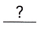Use the power of aproperty.
=Simplify.
(6 X 7)3 = (42)3,

Explanation:
Given 63 • 7when the variable bases are different and the
powers are the same, the bases are multiplied first,
Used the powers of product property,
(6 X 7)3 = (42)3.

Question 2.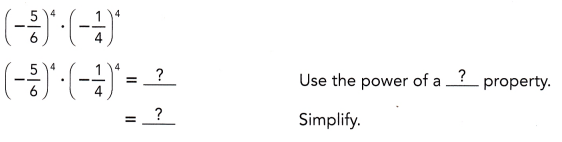(5/24)4,

Explanation:
Given (-5/6)4. (- 1/4)4 when the variable bases are different and the
powers are the same, the bases are multiplied first,
Used the powers of product and quotients of a property,
(-5/6 X -1/4)4 = (5/24)4.

Question 3.
(1.8)2 • (0.75)2
(1.8)2 • (0.75)2 =Use the power of aproperty.
=Simplify.
((1.8) • (0.75))2,

Explanation:
Given (1.8)2 • (0.75)2 when the variable bases are different and the
powers are the same, the bases are multiplied first,
Used the powers of product of a property,
((1.8) • (0.75))2.

Question 4.
p6 • q6
p6 • q6 =Use the power of aproperty.
=Simplify.
(p X q)6 ,

Explanation:
Given p6 • qwhen the variable bases are different and the
powers are the same, the bases are multiplied first,
Used the powers of product property,
(p X q)6 .

Question 5.
(3a)4 • (4b)4
(3a)4 • (4b)4 =Use the power of aproperty.
=Simplify.
(3a X 4b)4 ,

Explanation:
Given (3a)4 • (4b)4  when the variable bases are different and the
powers are the same, the bases are multiplied first,
Used the powers of product property we get (3aX 4b)4.

Question 6.
(-3y2)3 • $$\left(\frac{1}{12 y}\right)^{3}$$
$$\left(-\frac{y}{4}\right)^{3}$$,

Explanation:
Given (-3y2)3 • $$\left(\frac{1}{12 y}\right)^{3}$$ on simplification
(-y)2 X 3 • $$\left(\frac{1}{4 y}\right)^{3}$$ = (-y)6-3 • $$\left(\frac{1}{4}\right)^{3}$$ =
(-y)3 • $$\left(\frac{1}{4}\right)^{3}$$ = $$\left(-\frac{y}{4}\right)^{3}$$.

Question 7.
25 ÷ 45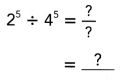$$\left(\frac{1}{2}\right)^{5}$$,

Explanation:
Given 25 ÷ 4as 4 can be written as (2 X 2) so 25 ÷ (2 X 2)5 upon simplification
we get 1/25 or $$\left(\frac{1}{2}\right)^{5}$$.

Question 8.
(-9)3 ÷ (-3)3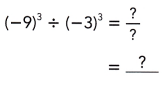(3)3,

Explanation:
Given(-9)3 ÷ (-3)as (-9) can be written as (-3 X 3) so (-3 X 3)3 ÷ (-3)3
on simplification we get (3)3.

Question 9.
x4 ÷ y4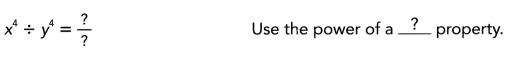$$\left(\frac{x}{y}\right)^{4}$$,

Explanation:
Given x4 ÷ y4 when the variable bases are different and the
powers are the same, the bases are simplified first as (x ÷ y)4 and solved.

Question 10.
(8p)5 ÷ (3q)5
(8p ÷ 3q)5,

Explanation:
Given (8p)5 ÷ (3q)5 when the variable bases are different and the
powers are the same, the bases are simplified first as (8p ÷ 3q)5 and solved.

Question 11.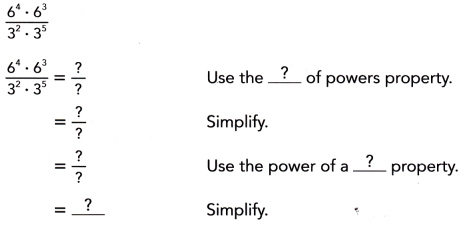(2)7,

Explanation: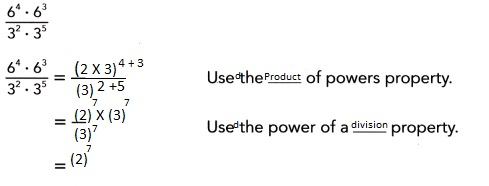Question 12.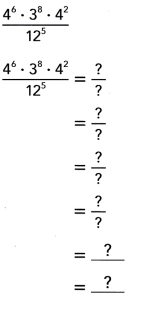(4)3 X (3)3,

Explanation: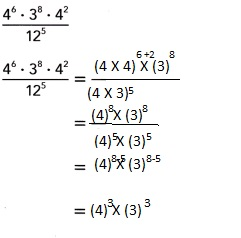Question 13.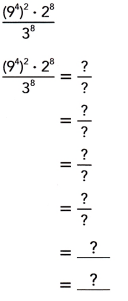(6)8,

Explanation: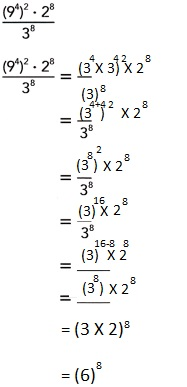Question 14.
$$\frac{2^{5} \cdot 3^{12} \cdot 2^{7}}{6^{7}}$$
(6)5,

Explanation:
Given $$\frac{2^{5} \cdot 3^{12} \cdot 2^{7}}{6^{7}}$$,
6 can be written as 2 X 3 so $$\frac{2^{5} \cdot 3^{12} \cdot 2^{7}}{({2 X 3})^{7}}$$,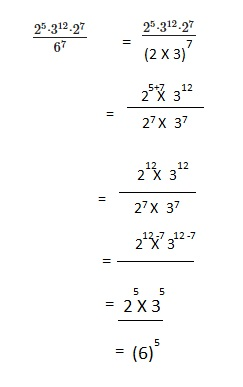Question 15.
$$\frac{\left(25^{3}\right)^{2} \cdot 7^{6}}{5^{6}}$$
(35)6,

Explanation:
Given $$\frac{\left(25^{3}\right)^{2} \cdot 7^{6}}{5^{6}}$$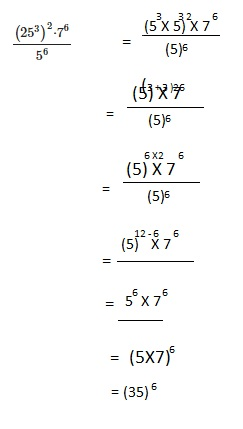### Math in Focus Course 3A Practice 1.4 Answer Key

Question 1.
54 • 64
(5 X 6)4 = (30)4,

Explanation:
Given 54 • 6when the variable bases are different and the
powers are the same, the bases are multiplied first,
Used the powers of product property,
(5 X 6)4 = (30)4.

Question 2.
5.43 • 4.53
(5.4 X 4.5)3 = (24.3)3,

Explanation:
Given 5.43 • 4.5when the variable bases are different and the
powers are the same, the bases are multiplied first,
Used the powers of product property,
(5.4 X 4.5)3 = (24.3)3.

Question 3.
25 • 105
(2 X 10)5 = (20)5,

Explanation:
Given 25 • 10when the variable bases are different and the
powers are the same, the bases are multiplied first,
Used the powers of product property,
(2 X 10)5 = (20)5.

Question 4.
a3 • b3
(a X b)3 = (ab)3,

Explanation:
Given a3 • bwhen the variable bases are different and the
powers are the same, the bases are multiplied first,
Used the powers of product property,
(a X b)3 = (ab)3.

Question 5.
(2x)5 • (3y)5
(2x X 3y)5 = (6xy)5,

Explanation:
Given (2x)5 • (3y)when the variable bases are different and the
powers are the same, the bases are multiplied first,
Used the powers of product property,
(2x X3y)5 = (6xy)5.

Question 6.
(2.5a)6 • (1.6b)6
(2.5a X 1.6b)6 = (4ab)6,

Explanation:
Given (2.5a)6 • (1.6b)when the variable bases are different and the
powers are the same, the bases are multiplied first,
Used the powers of product property,
(2.5a X 1.6b)6 = (4ab)6.

Question 7.
$$\left(-\frac{1}{3}\right)^{4} \cdot\left(-\frac{2}{5}\right)^{4}$$
$$\left(\frac{2}{15}\right)^{4}$$,

Explanation:
Given $$\left(-\frac{1}{3}\right)^{4} \cdot\left(-\frac{2}{5}\right)^{4}$$
when the variable bases are different and the powers are the same,
the bases are multiplied first,Used the powers of product property,
$$\left(\frac{-1 X -2}{3X 5}\right)^{4}$$ = $$\left(\frac{2}{15}\right)^{4}$$.

Question 8.
92 ÷ 32
(3)2,

Explanation:
Given (9)2 ÷ (3)as 9 = 3 X 3 can be written as (32)2 so (3)4 ÷ (3)2
on simplification we get (3)4-2 = (3)2.

Question 9.
106 ÷ 56
(2)6,

Explanation:
Given (10)6 ÷ (5)as 10 can be written as (5 x 2)6 so (5)6 .(2)6 ÷ (5)6
on simplification we get (2)6.

Question 10.
2.87 ÷ 0.77
(4)7,

Explanation:
Given (2.8)7 ÷ (0.7)as 2.8 ÷ 0.7 = 4 so can be written as (4)7 .

Question 11.
152 ÷ 252
$$\left(\frac{3}{5}\right)^{2}$$,

Explanation:
Given 152 ÷ 252, 152 can be written as (5 X 3)2 and 252  as (5 X 5)2  ,
upon simplification we get $$\left(\frac{3}{5}\right)^{2}$$.

Question 12.
7.29 ÷ 2.49
(3)9,

Explanation:
Given (7.2)9 ÷ (2.4)as 7.2 ÷ 2.4 = 3, so can be written as (3)9.

Question 13.
(3.2x)9 ÷ (1.1y)9
(3.2x ÷ 1.1y)9,

Explanation:
Given (3.2x)9 ÷ (1.1y)9 when the variable bases are different and the
powers are the same, the bases are multiplied first,
Used the powers of product property (3.2x ÷ 1.1y)9.

Question 14.
(-6)8 ÷ (-2)8
(3)8,

Explanation:
Given (-6)8 ÷ (-2)as -6 can be written as (-2 x 3)8 so (-2)8 .(3)8 ÷ (-2)8
on simplification we get (3)8.

Question 15.
s5 ÷ r5
$$\left(\frac{s}{r}\right)^{5}$$,

Explanation:
Given s5 ÷ r5 the variable bases are different and the
powers are the same, the bases are divided first,upon simplification we get
$$\left(\frac{s}{r}\right)^{5}$$.

Question 16.
(3a)6 ÷ (2b)6
$$\left(\frac{3a}{2b}\right)^{6}$$,

Explanation:
Given (3a)6 ÷ (2b)6 the variable bases are different and the
powers are the same, the bases are divided first, upon simplification we get
$$\left(\frac{3a}{2b}\right)^{6}$$.

Question 17.
(h2k5)4
h8k20,

Explanation:
Given (h2k5)4 when the variable bases are different and the
powers are the same,Used the powers of product and
quotients of a property we get h2X 4 X k5X4 = h8k20.

Question 18.
$$\left(\frac{32 m^{6}}{4 n^{4}}\right)^{2}$$
$$\left(\frac{8 m^{6}}{4n^{4}}\right)^{2}$$,

Explanation:
Given $$\left(\frac{32 m^{6}}{4 n^{4}}\right)^{2}$$ upon simplification
we get $$\left(\frac{8 m^{6}}{4n^{4}}\right)^{2}$$.

Question 19.
$$\frac{9^{2} \cdot 9^{7}}{3^{5} \cdot 3^{4}}$$
(3)9,

Explanation:
Given $$\frac{9^{2} \cdot 9^{7}}{3^{5} \cdot 3^{4}}$$
9 can be written as $$\frac{3 X 3 ^{2} \cdot 3 X 3^{7}}{3^{5} \cdot 3^{4}}$$
when bases are same powers are added $$\frac{3^{2+2+7+7}}{3^{5+4}}$$ =
$$\frac{3^{18}}{3^{9}}$$ on further simplification we get (3)18-9 = (3)9.

Question 20.
$$\frac{6^{5} \cdot 2^{3} \cdot 6^{4}}{12^{3}}$$
(6)6,

Explanation:
Given$$\frac{6^{5} \cdot 2^{3} \cdot 6^{4}}{12^{3}}$$ upon simplification
$$\frac{6^{5+4} \cdot 2^{3} }{6 X 2^{3}}$$ = $$\frac{6^{9} \cdot 2^{3} }{(6 X 2)^{3}}$$=
(6)9-3 = (6)6.

Question 21.
$$\frac{\left(5^{4}\right)^{2} \cdot 6^{8}}{10^{8}}$$
(3)8,

Explanation:
Given $$\frac{\left(5^{4}\right)^{2} \cdot 6^{8}}{10^{8}}$$ as denominator
10 can be written as 5 X 2 so $$\frac{\left(5\right)^{8} \cdot 6^{8}}{(5 X 2)^{8}}$$ we get
$$\frac{6^{8}}{2^{8}}$$ = $$\frac{(2 X 3)^{8}}{2^{8}}$$ = (3)8.

Question 22.
$$\frac{\left(6^{3}\right)^{3} \cdot 4^{9}}{8^{9}}$$
(3)9,

Explanation:
Given $$\frac{\left(6^{3}\right)^{3} \cdot 4^{9}}{8^{9}}$$ as denominator
8 can be written as 4 X 2 so  we get
$$\frac{\left((2 X 3)^{3}\right)^{3} \cdot 4^{9}}{(4 X 2)^{9}}$$ = (3)9.

Question 23.
$$\frac{24^{9}}{4^{3} \cdot 6^{2} \cdot 4^{6}}$$
(6)7,

Explanation:
Given $$\frac{24^{9}}{4^{3} \cdot 6^{2} \cdot 4^{6}}$$ as
24 in numerator can be written as 4 X 6 so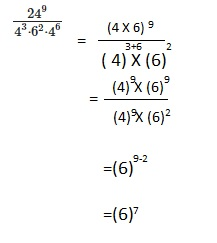Question 24.
$$\frac{9^{12}}{\left(3^{3}\right)^{3} \cdot 3^{3}}$$
(3)12,

Explanation: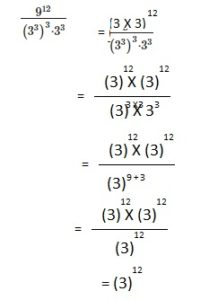Question 25.
Math Joural Charles thinks that a3 • b3 = ab6. Is he correct? Why?
No, Charles is incorrect,

Explanation:
a3 • b3 ≠ ab6 it should be (ab)3 as when the variable bases are different and the
powers are the same, the bases are multiplied first,
Used the powers of product property we get (aX b)3 ≠ ab6.

At the beginning of January, Mr. Howard gives his niece $1 to start a savings account. For each month that she can triple the amount in the account, Mr. Howard will double the amount in the account at the end of each month. How much does Mr. Howard’s niece have in her account at the beginning of May? Answer:$7,776 Mr. Howard’s niece have in her account at the beginning of May,
Given at the beginning of January, Mr. Howard gives his niece $1 to start a savings account. For each month that she can triple the amount in the account, Mr. Howard will double the amount in the account at the end of each month. So at the beginning of January it is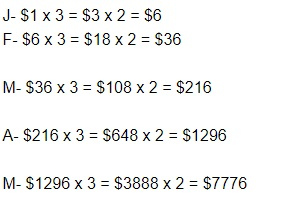therefore,$7,776 Mr. Howard’s niece have in her account at the beginning of May.